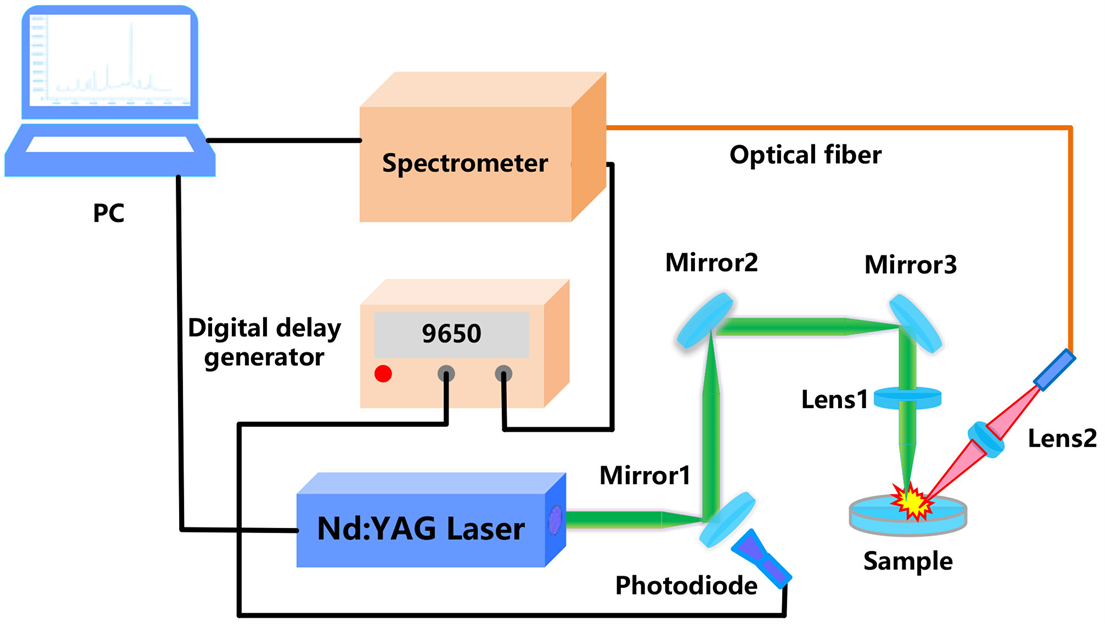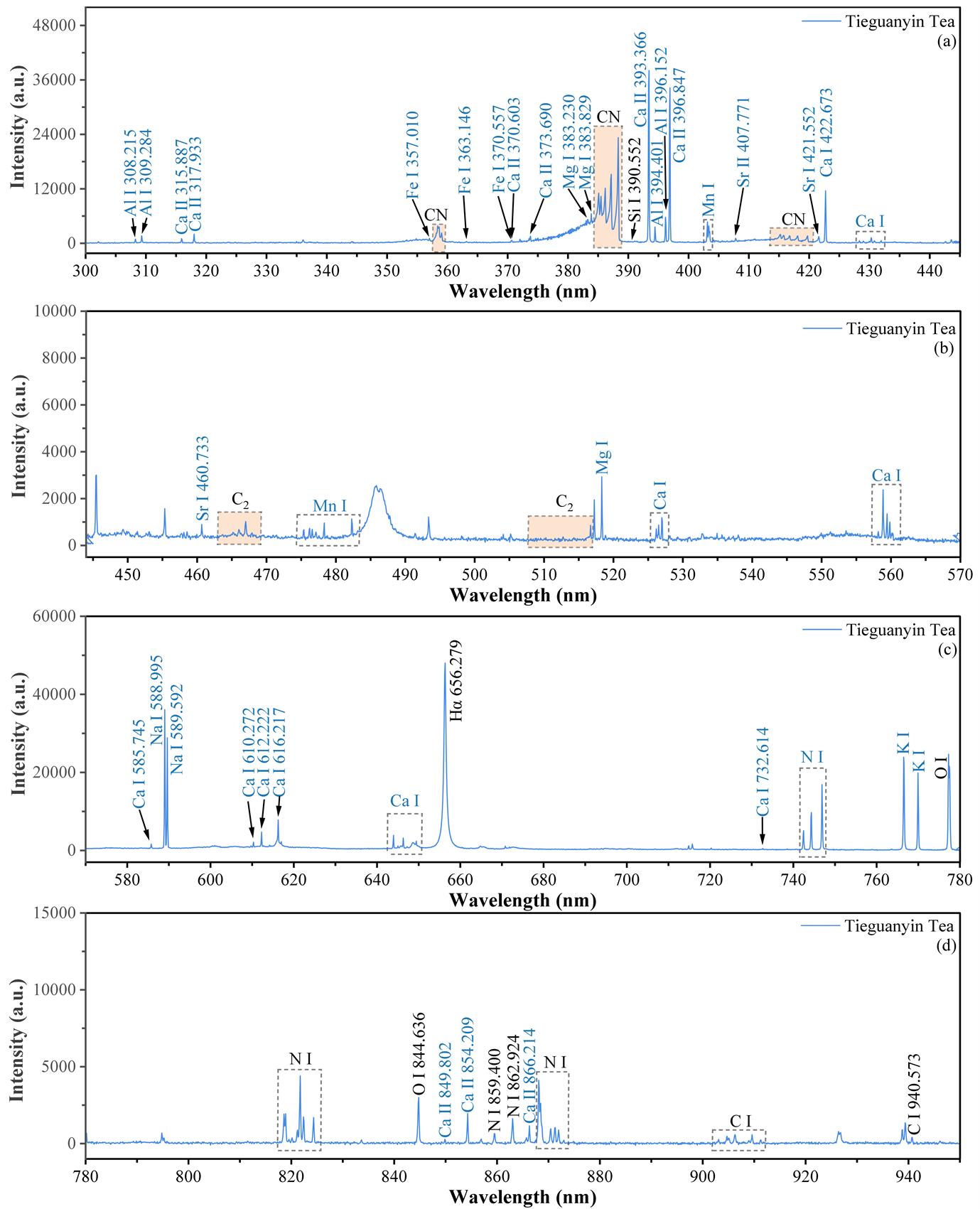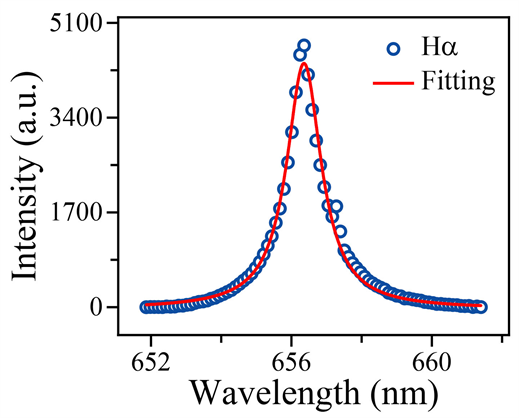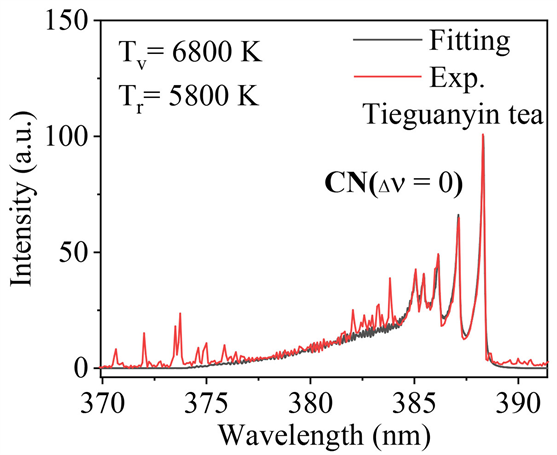#### 期刊菜单

Study on the Characteristics of Laser-Induced Plasma in Tieguanyin Tea

Abstract: China is the hometown and birthplace of tea. Nowadays, tea culture has spread to all over the world and tea is the second largest beverage after water. In this study, the elemental composi-tion of Tieguanyin tea was studied by laser-induced breakdown spectroscopy (LIBS). Ca, Na, Mg, K, Fe, Cu, Zn, Mn, Sr, Al, Li and Ba were found in Tieguanyin tea. The spectral lines of CN and C2 were also detected. Then, the plasma characteristics of Tieguanyin tea of laser-induction were researched. The electron density (Ne) was 1.44 × 1017 cm−3 by using the Lorentz fitting of the Hα line. The Sa-ha-Boltzmann plot was established, and the plasma temperature (T) was calculated to be 8161 K. Finally, LIFBASE software was used to simulate CN ( △v=0 ) molecule, and the vibration tempera-ture (Tv) and rotation temperature (Tr) were obtained as 6800 K and 5800 K, respectively. In the experiment, the three temperatures met the relationship T > Tv > Tr, which was consistent with the actual situation of plasma evolution over time.

1. 引言

2. LIBS实验装置Figure 1. Schematic diagram of LIBS device

3. 结果与讨论

3.1. 铁观音茶叶的LIBS光谱图Figure 2. LIBS spectra of Tieguanyin tea

3.2. 等离子体特性的研究

1) 等离子体中各元素含量与样品中各元素含量相同；

2) 局域热力学平衡；

3) 光学薄(主要电离过程来自于热电子的碰撞激发过程)。

${I}_{\lambda }^{ki}={A}_{ki}{n}_{k}^{s}={n}^{s}{A}_{ki}{g}_{k}\frac{{\text{e}}^{-\frac{{E}_{k}}{kT}}}{{U}_{s}\left(T\right)}.$ (1)

${\stackrel{¯}{I}}_{\lambda }^{ki}=F{n}^{s}{A}_{ki}{g}_{k}\frac{{\text{e}}^{-\frac{{E}_{k}}{kT}}}{{U}_{s}\left(T\right)}=F{n}^{p}{C}_{s}{A}_{ki}{g}_{k}\frac{{\text{e}}^{-\frac{{E}_{k}}{kT}}}{{U}_{s}\left(T\right)}.$ (2)

$y=\mathrm{ln}\frac{{\stackrel{¯}{I}}_{\lambda }^{ki}}{{A}_{ki}{g}_{k}},\text{}x={E}_{k},\text{}m=-\frac{1}{kT}\text{,}{q}_{s}=\mathrm{ln}\frac{F{n}^{p}{C}_{s}}{{U}_{s}\left(T\right)}.$ (3)

$y=mx+{q}_{s}.$ (4)

${C}_{s}=\frac{{U}_{s}\left(T\right){\text{e}}^{{q}_{s}}}{F{n}^{p}}.$ (5)

$\underset{{s}^{\prime }}{\sum }{C}_{{s}^{\prime }}=\frac{1}{F{n}^{p}}\underset{{s}^{\prime }}{\sum }{U}_{{s}^{\prime }}\left(T\right){q}_{{s}^{\prime }}=1.$ (6)

$F{n}^{p}=\underset{{s}^{\prime }}{\sum }{U}_{{s}^{\prime }}\left(T\right){q}_{{s}^{\prime }}.$ (7)

${C}_{s}=\frac{{U}_{s}\left(T\right){\text{e}}^{{q}_{s}}}{\underset{{s}^{\prime }}{\sum }{U}_{{s}^{\prime }}\left(T\right){q}_{{s}^{\prime }}}.$ (8)

$\frac{{C}_{s}^{a}}{{C}_{s}^{b}}=\frac{{U}_{s}^{a}\left(T\right){\text{e}}^{{q}_{s}^{a}}}{{U}_{s}^{b}\left(T\right){\text{e}}^{{q}_{s}^{b}}}\text{.}$ (9)

$\frac{{n}^{II}}{{n}^{I}}=\frac{{\left(2\pi {m}_{e}kT\right)}^{3/2}}{{n}_{e}{h}^{3}}\frac{2{U}^{II}\left(T\right)}{{U}^{I}\left(T\right)}{\text{e}}^{-\frac{{E}_{\text{ion}}}{kT}}\text{.}$ (10)

${I}_{jh}^{II}={n}^{II}{A}_{jh}^{II}{g}_{j}^{II}\frac{{\text{e}}^{-\frac{{E}_{j}^{II}}{kT}}}{{U}^{II}\left(T\right)}={A}_{jh}^{II}{g}_{j}^{II}\frac{{\text{e}}^{-\frac{{E}_{j}^{II}}{kT}}}{{U}^{I}\left(T\right)}\frac{{n}^{I}}{{n}_{e}}\frac{2{\left(2\pi {m}_{e}\right)}^{3/2}}{{h}^{3}}{\left(kT\right)}^{3/2}{\text{e}}^{-\frac{{E}_{\text{ion}}}{kT}}\text{.}$ (11)

$\mathrm{ln}\left(\frac{{\stackrel{¯}{I}}_{jh}^{II}}{{A}_{jh}^{II}{g}_{j}^{II}}\right)=\mathrm{ln}\left(\frac{F{n}^{p}{C}^{I}}{{U}^{I}\left(T\right)}\right)+\mathrm{ln}\left(\frac{2{\left(2\pi {m}_{e}kT\right)}^{3/2}}{{n}_{e}{h}^{3}}\right)-\left(\frac{{E}_{\text{ion}}+{E}_{j}^{II}}{kT}\right)\text{.}$ (12)

${x}^{*}=\left\{\begin{array}{l}{E}_{k}\text{}原子线\\ {E}_{j}+{E}_{\text{ion}}\text{}离子线\end{array}$ (13)

${y}^{*}=\left\{\begin{array}{l}\mathrm{ln}\frac{{\stackrel{¯}{I}}_{\lambda }^{ki}}{{A}_{ki}{g}_{k}}\text{}原子线\\ \mathrm{ln}\left(\frac{{\stackrel{¯}{I}}_{jh}^{II}}{{A}_{jh}^{II}{g}_{j}^{II}}\right)-\mathrm{ln}\left(\frac{2{\left(2\pi {m}_{e}kT\right)}^{3/2}}{{n}_{e}{h}^{3}}\right)\text{}离子线\end{array}$ (14)

$\Delta E=\frac{z{e}^{2}}{4\pi {\epsilon }_{0}{{\rho }^{\prime }}_{D}},$ (15)

${\epsilon }_{0}$ (F m−1)为真空中介电常数， ${{\rho }^{\prime }}_{D}$ (nm)为德拜半径。德拜半径

${{\rho }^{\prime }}_{D}=\sqrt{\frac{{\epsilon }_{0}kT}{{e}^{2}\left({n}_{e}+\underset{z}{\sum }{z}^{2}{n}_{z}\right)}},$ (16)

$\Delta E=\frac{z{e}^{3}}{4\pi }\sqrt{\frac{{n}_{e}}{{\epsilon }_{0}^{3}kT}}.$ (17)

${n}_{e}\left({\text{cm}}^{-3}\right)={10}^{17}{\left(\text{FWHA}\left(\text{nm}\right)/0.549\right)}^{1.4713}$ (19)Figure 3. Lorentz fitting of Hα 656.361 nmFigure 4. Saha-Boltzmann plot of Ca element in Tieguanyin tea

3.3. CN分子的振动温度和转动温度Figure 5. Simulation and experimental results of CN ( $\Delta \nu =\text{0}$ ) molecules in Tieguanyin tea

4. 结论

  Xu, Y.Q., Chen, S.Q., Shen, D.Y. and Yin, J.F. (2011) Effects of Chemical Components on the Amount of Green Tea Cream. Agricultural Sciences in China, 10, 969-974. https://doi.org/10.1016/S1671-2927(11)60083-7  Sun, J.W., Hu, G.R., Liu, K.K., et al. (2019) Potential Exposure to Metals and Health Risks of Metal Intake from Tieguanyin Tea Production in Anxi, China. Environmental Geochemistry and Health, 41, 1291-1302. https://doi.org/10.1007/s10653-018-0212-y  Cao, Q.Q., Fu, Y.Q., Wang, J.Q., et al. (2021) Sensory and Chemical Characteristics of Tieguanyin Oolong Tea after Roasting. Food Chemistry: X, 12, Article ID: 100178. https://doi.org/10.1016/j.fochx.2021.100178  Wu, H., Hou, D.Y., Hui, R.H., et al. (2013) Lead Chrome Green Determination in Tea by Inductive Coupled Plasma Atomic Emission Spectrometry. Journal of Anshan Normal Univer-sity, 15, 41-43.  Tokalioglu, S. (2012) Determination of Trace Elements in Commonly Consumed Medicinal Herbs by ICP-MS and Multivariate Analysis. Food Chemistry, 134, 2504-2508. https://doi.org/10.1016/j.foodchem.2012.04.093  Li, X.L., Zhou, R.Q., Sun, C.J., et al. (2017) Detection of Lead Chrome Green Illegally Added in Tea Based on Confocal Raman Spectroscopy. Spectroscopy and Spectral Analysis, 37, 461-466.  Li, X.L., Xu, K.W., Zhang, Y.Y., Sun, C.J. and He, Y. (2017) Optical Determination of Lead Chrome Green in Green Tea by Fourier Transform Infrared (FT-IR) Transmission Spectroscopy. PLOS ONE, 12, e0169430. https://doi.org/10.1371/journal.pone.0169430  Shi, J.E., Hua, L., Li, Y.Q., et al. (2019) Determination of Lead Chrome Green in Tea by Ion Chromatography and Spectrophotometry. Journal of Food Safety and Quality, 10, 1534-1540.  Guo, X.H., Zhao, P., Wu, Y.Q., et al. (2022) Application of XRF and ICP-MS in Elements Content Determinations of Tieguanyin of Anxi and Hua’an County, Fujian Province. Spectroscopy and Spectral Analysis, 42, 3124-3129.  Nasrazadani, S. and Namduri, H. (2006) Study of Phase Transformation in Iron Oxides Using Laser Induced Breakdown Spectroscopy. Spectrochimica Acta Part B: Atomic Spectroscopy, 61, 565-571. https://doi.org/10.1016/j.sab.2006.04.001  刘烨坤. 激光诱导击穿土壤重金属光谱增强及定量分析方法研究[D]: [硕士学位论文]. 太原: 中北大学, 2022.  Zhang, Q.H., Liu, Y.Z., Yin, W.Y., et al. (2020) The in situ De-tection of Smoking in Public Area by Laser-Induced Breakdown Spectroscopy. Chemosphere, 242, Article ID: 125184. https://doi.org/10.1016/j.chemosphere.2019.125184  Ding, Y., Xia, G.Y., Ji, H.W. and Xiong, X. (2019) Ac-curate Quantitative Determination of Heavy Metals in Oily Soil by Laser Induced Breakdown Spectroscopy (LIBS) Combined with Interval Partial Least Squares (IPLS). Analytical Methods, 11, 3657-3664. https://doi.org/10.1039/C9AY01030K  Tian, Y., Chen, Q., Lin, Y.Q., et al. (2021) Quantitative Determination of Phosphorus in Seafood Using Laser-Induced Breakdown Spectroscopy Combined with Machine Learning. Spectro-chimica Acta Part B: Atomic Spectroscopy, 175, Article ID: 106027. https://doi.org/10.1016/j.sab.2020.106027  Teng, G., Wang, Q.Q., Yang, H.F., et al. (2020) Pathological Iden-tification of Brain Tumors Based on the Characteristics of Molecular Fragments Generated by Laser Ablation Combined with a Spiking Neural Network. Biomedical Optics Express, 11, 4276-4289. https://doi.org/10.1364/BOE.397268  Singh, J.P. and Thakur, S.N. (2020) Laser-Induced Breakdown Spec-troscopy. Elsevier, Amsterdam.  陈宇. 基于激光光谱技术的大气硫化物在线探测[D]: [硕士学位论文]. 南京: 南京信息工程大学, 2022.  何亚雄, 周文琦, 柯川, 等. 激光诱导击穿光谱技术在气体检测中的研究综述[J]. 光谱学与光谱分析, 2021, 41(9): 2681-2687.  Dietz, T., Kohns, P. and Ankerhold, G. (2018) Diagnostics and Simulations of Molecular Formation in Laser-Induced Plasmas. Spectrochimica Acta Part B: Atomic Spectroscopy, 148, 51-59. https://doi.org/10.1016/j.sab.2018.06.007  Kramida, A., Ralchenko, Y., Reader, J., et al. (2022) NIST Atomic Spectra Database (Version 5.10). National Institute of Standards and Technology, Gaithersburg.  Gondal, M.A., Habibullah, Y.B., Baig, U. and Oloore, L.E. (2016) Direct Spectral Analysis of Tea Samples Using 266nm UV Pulsed Laser-Induced Breakdown Spectroscopy and Cross Validation of LIBS Results with ICP-MS. Talanta, 152, 341-352. https://doi.org/10.1016/j.talanta.2016.02.030  Rehan, I., Gondal, M.A., Aldakheel, R.K., et al. (2022) Determination of Nutritional and Toxic Metals in Black Tea Leaves Using Calibration Free LIBS and ICP: AES Tech-nique. Arabian Journal for Science and Engineering, 47, 7531-7539. https://doi.org/10.1007/s13369-021-06233-y  Lu, X., Liu, Y.Z., Zhang, Q.H. and Li, L. (2020) Study on Tea Harvested in Different Seasons Based on Laser-Induced Breakdown Spectroscopy. Laser Physics Letters, 17, Article ID: 015701. https://doi.org/10.1088/1612-202X/ab5c23  CabalíN, L.M., Delgado, T., Garcia-Gomez, L. and Laserna, J.J. (2020) Considerations on Formation Mechanisms of Emitting Species of Organic and C-Containing Inorganic Com-pounds in CO2 Atmosphere Using Laser-Induced Breakdown Spectroscopy as a Strategy for Detection of Molecular Solids. Spectrochimica Acta Part B: Atomic Spectroscopy, 169, Article ID: 105869. https://doi.org/10.1016/j.sab.2020.105869  Zhang, Q., Liu, Y., Yin, W., et al. (2020) The Online Detection of Carbon Isotopes by Laser-Induced Breakdown Spectroscopy. Journal of Analytical Atomic Spectrometry, 35, 341-346. https://doi.org/10.1039/C9JA00384C  Zhangcheng, Y.Z., Liu, Y.Z., Saleem, S., et al. (2020) Online in situ Detection and Rapid Distinguishing of Saffron. Journal of Laser Applications, 32, Article ID: 032020. https://doi.org/10.2351/7.0000137  Civis, M., Civis, S., Sovova, K., et al. (2011) Laser Ablation of FOX-7: Proposed Mechanism of Decomposition. Analytical Chemistry, 83, 1069-1077. https://doi.org/10.1021/ac1028769  Wang, J.M., Zheng, P.C., Liu, H.D. and Fang, L. (2016) Classification of Chinese Tea Leaves Using Laser-Induced Breakdown Spectroscopy Combined with the Discriminant Analysis Method. Analytical Methods, 8, 3204-3209. https://doi.org/10.1039/C5AY03260A  Portnov, A., Rosenwaks, S. and Bar, I. (2003) Emission following Laser-Induced Breakdown Spectroscopy of Organic Compounds in Ambient Air. Applied Optics, 42, 2835-2842. https://doi.org/10.1364/AO.42.002835  Yan, Y.H., Liu, Y.Z., Zhang, Q.H. and Ding, P.F. (2020) Correlation between Laser-Induced Plasma Temperature and CN Radical Molecule Emission during Tree Burning. Optik, 224, Article ID: 165670. https://doi.org/10.1016/j.ijleo.2020.165670  Ciucci, A., Corsi, M., Palleschi, V., et al. (1999) New Procedure for Quantitative Elemental Analysis by Laser-Induced Plasma Spectroscopy. Applied Spectroscopy, 53, 960-964. https://doi.org/10.1366/0003702991947612  Tognoni, E., Cristoforetti, G., Legnaloli, S., et al. (2007) A Nu-merical Study of Expected Accuracy and Precision in Calibration-Free Laser-Induced Breakdown Spectroscopy in the Assumption of Ideal Analytical Plasma. Spectrochimica Acta Part B: Atomic Spectroscop, 62, 1287-1302. https://doi.org/10.1016/j.sab.2007.10.005  Gigosos, M.A., Gonzalez, M.A. and Cardenoso, V. (2003) Com-puter Simulated Balmer-α, -β and -γ Stark Line Profiles for Non-Equilibrium Plasmas Diagnostics. Spectrochimica Acta Part B: Atomic Spectroscop, 58, 1489-1504. https://doi.org/10.1016/S0584-8547(03)00097-1  Gaft, M., Nagli, L., Gornushkin, I. and Raichlin, Y. (2020) Review on Recent Advances in Analytical Applications of Molecular Emission and Modelling. Spectrochimica Acta Part B: Atomic Spectroscopy, 173, Article ID: 105989. https://doi.org/10.1016/j.sab.2020.105989